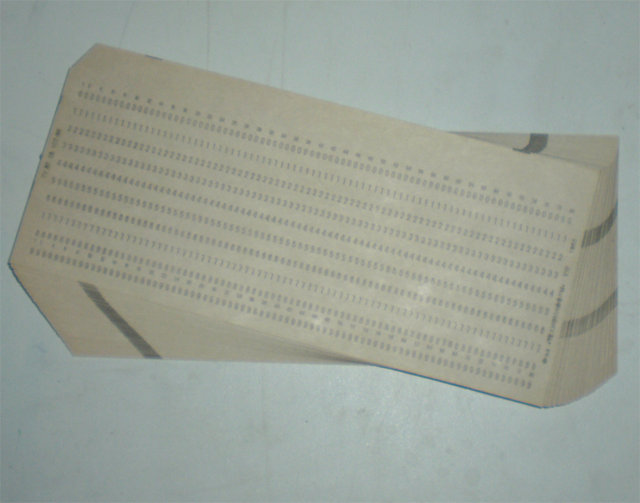## Saturday, January 08, 2011

### towards Problem Compilers

First programmers wrote in machine code and assemblers simplified this task significantly by letting them give algorithms at a higher level. I still find stacks of punch cards like below in my St.Petersburg homeWouldn't it be great if we could extend this idea further and have the computer compile the problem into machine code?

Actually, we already have such tools restricted to various versions of "problem language". For instance if you express your problem in terms of integer optimization, you can use integer programming tools.

Another language is the language of "graphical models". In the most general formulation, you specify meaning of operations + and *, that obey distributive law, set of x variables, set of functions f, and ask computer to find the following

$$\bigoplus_{x_1,x_2,\ldots} \bigotimes_f f(x)$$

This formulation has a natural "interactions graph" associated with it, and a computer can find an algorithm to solve this problem using tree decomposition if interactions between f functions are not too complex

However, this is still suboptimal because the algorithm designer needs to pick variables x and functions f, and this choice is fairly important. For instance, for minimum weight Steiner Tree, "natural" variables of the problem don't give a good factorization, and you need a smart designer to figure out a good reparameterization of the problem, like here.

As it stands right now, if a designer gives a good parameterization of the problem, a computer can solve it. Can we train a computer to find a parameterization?

I don't know the answer, but one potential approach to finding parameterizations is described in A new look at Generalized Distributive Law. They formulate the problem of optimal decomposition as that of search at the level of individual instantiations of x, so theoretically, this approach can discover optimal parameterization of the problem.